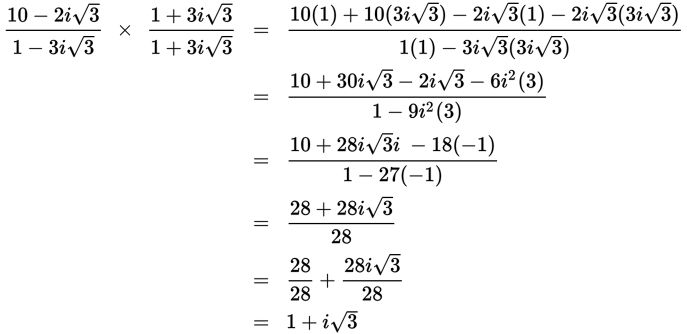# SAT Math Multiple Choice Question 950: Answer and Explanation

### Test Information

Question: 950

2. Which of the following is equivalent to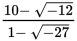? (Note: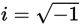)

• A.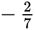• B.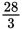• C.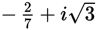• D.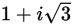Explanation:

D

Difficulty: Hard

Category: Additional Topics in Math / Imaginary Numbers

Strategic Advice: Resist the urge to rationalize the denominator right away. Before doing this, you should remove the negative signs from under the radicals.

Getting to the Answer: Rewrite the number under each radical as a product of -1 and convenient factors of the number. Then simplify if possible: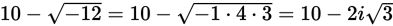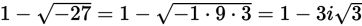Next, multiply the numerator and denominator of the resulting expression by the conjugate of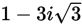and simplify: